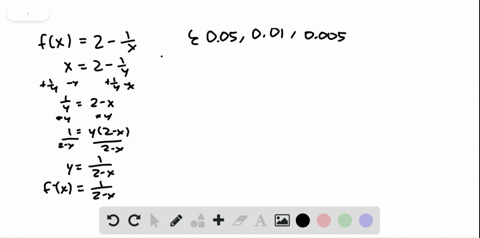Enroll in one of our FREE online STEM bootcamps. Join today and start acing your classes!View Bootcamps### Finding a $\delta$ for a Given $\varepsilon$ Repe…

04:52University of California, Berkeley
Problem 37

# Finding a $\delta$ for a Given $\varepsilon$ The graph of$f(x)=2-\frac{1}{x}$is shown in the figure. Find $\delta$ such that if $0<|x-1|<\delta$ then $|f(x)-1|<0.1$

## Discussion

You must be signed in to discuss.

## Video Transcript

okay for my figure, we knew that one is a good one. And that doctor value of our function when it won his last in 0.1. This implies that they'll 0.1. Okay, One. Okay, so we know our function is to monitor one overact we calculated in verse. So the finest invest. Okay, Was that wise? Because the two letters one overact is the fact two from both sides get wine money too. You could make it a one overact that mean, huh? Negative. Or the inverse effect is he could be won over human effect. I'm plugging us into 0.9 you got this is equal to one over to manage 10.9. The use of 10 over 11. If we do the same for 1.1, get one over by this 1 21 if you want overnight. Therefore, as after a while, you go back one, Elsa. But that function, but it's one. Is that your 0.1? Yeah. 10 over 11 is lots of extras. An overnight. So delta is equal to the minimum of one minus 10 over 11 or 10 overlying might one. And that is equal to one over 11